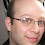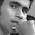## Sunday, January 15, 2012

### C11 - Generic Selections

C99 introduced type-generic macros defined in the new `tgmath.h` header. These are macros that expand to the appropriate function based on the type of the provided argument. For example, their are 6 different arc cosine functions: 3 for the different real floating point types and 3 for the different complex floating point types. If you `#include <tgmath.h>`, a macro with the name `acos` is exposed which will expand to a call to one of the 6 functions based on the argument type.

The type-generic mechanism employed to make this work was not exposed or made available to the programmer but was instead left up to implementation magic. C11 introduces the generic selection expression which provides a mechanism to make compile-time choices based on type allowing type-generic macros to be created using standard C constructs.

In this post I will introduce and explore some of the applications of the new generic selection.

### Overview

Generic selection is implemented with a new keyword: `_Generic`. The syntax is similar to a simple switch statement for types:
`    _Generic( 'a', char: 1, int: 2, long: 3, default: 0)`
evaluates to 2 (character constants are ints in C).

The use of `_Generic` can be abstracted in a macro:
`    #define type_idx(T) _Generic( (T), char: 1, int: 2, long: 3, default: 0)`
So that `type_idx('a')` evaluates to 2 and `type_idx("a")` evaluates to 0.

### Details

• A generic selection consists of a controlling expression (which is not evaluated) and one or more, comma-separated, generic associations.
• Each generic association consists of a type-name (or `default`) and a result expression separated by a colon.
• The result of the generic selection expression is the result expression of the corresponding compatible type provided in the matching generic association.
• If none of the provided types are compatible with the type of the controlling expression, a default selection is used if provided, otherwise the construct is erroneous.
• The order of the generic associations in the list is inconsequential; no more than one compatible type may be provided in a generic selection so there is never more than one case that could match.
• The type name in a generic association must be with a complete, non-VLA, type.
• The controlling expression can be any expression.
• The types of the result expressions can vary between the provided generic associations.

### Examples

#### Type Generic Macros

The driving force behind `_Generic` is to provide a pseudo type-polymorphism mechanism. For example, the `acos` macro defined in `tgmath.h` could be implemented as:
```#define acos(X) _Generic((X), \
long double complex: cacosl, \
double complex: cacos, \
float complex: cacosf, \
long double: acosl, \
float: acosf, \
default: acos \
)(X)
```
This works well for single-argument functions but becomes more complex for multiple-argument functions. For example, `tgmath.h` provides a `pow` macro that expands to one of 6 functions but it accepts two arguments, both of which must be considered when determining the version of the function to call. A possible implementation for the `pow` macro is (empty lines added for readability):
```#define pow(x, y) _Generic((x), \
long double complex: cpowl, \

double complex: _Generic((y), \
long double complex: cpowl, \
default: cpow), \

float complex: _Generic((y), \
long double complex: cpowl, \
double complex: cpow, \
default: cpowf), \

long double: _Generic((y), \
long double complex: cpowl, \
double complex: cpow, \
float complex: cpowf, \
default: powl), \

default: _Generic((y), \
long double complex: cpowl, \
double complex: cpow, \
float complex: cpowf, \
long double: powl, \
default: pow), \

float: _Generic((y), \
long double complex: cpowl, \
double complex: cpow, \
float complex: cpowf, \
long double: powl, \
float: powf, \
default: pow) \
)(x, y)
```

#### Printing values generically

Similar to selecting the name of a function to call based on the argument type, we can select, for example, a `printf` format specifier based on type. This allows the creation of a macro that can print any type of value that `printf` supports without having to specify the type explicitly in the call:
```#define printf_dec_format(x) _Generic((x), \
char: "%c", \
signed char: "%hhd", \
unsigned char: "%hhu", \
signed short: "%hd", \
unsigned short: "%hu", \
signed int: "%d", \
unsigned int: "%u", \
long int: "%ld", \
unsigned long int: "%lu", \
long long int: "%lld", \
unsigned long long int: "%llu", \
float: "%f", \
double: "%f", \
long double: "%Lf", \
char *: "%s", \
void *: "%p")

#define print(x) printf(printf_dec_format(x), x)
#define printnl(x) printf(printf_dec_format(x), x), printf("\n");
```
We can then print values like so:
```    printnl('a');    // prints "97" (on an ASCII system)
printnl((char)'a');  // prints "a"
printnl(123);    // prints "123"
printnl(1.234);      // prints "1.234000"
```
Note that since `'a'` is an `int` and we set `int` up to use the `"%d"` format specifier, we have to cast it to a `char` if we want to print out the letter.

`    printnl("abc");    // error on clang`
This produces an error on clang. The problem is that `"abc"` has type `char ` (3 plus the nul terminator) which is not compatible with the `char *` type in our macro. An array of type is converted to a pointer to type except in certain cases as described in §6.3.2.1p3:

Except when it is the operand of the sizeof operator, the _Alignof operator, or the
unary & operator, or is a string literal used to initialize an array, an expression that has
type ‘‘array of type’’ is converted to an expression with type ‘‘pointer to type’’ that points
to the initial element of the array object and is not an lvalue.

This clause makes no mention of `_Generic` so one would assume that the conversion should occur in this case, this may be a defect in clang.

So how do we handle it? We can't use `char []` as the type name because `char []` is not a complete type. If we wish to use this macro with a string literal (at least on clang), we will need a cast:
`    printnl((char *)"abc");    // ok`
Another problem occurs when we try to print a variable of qualified type:
```    const int ci = 123;
printnl(ci);     // error
```
The problem is that `const int` is not compatible with `int` so there is no valid generic association. We can either cast `ci` to `char *` or expand our macro to handle `const` types (and possibly `volatile` types and `const volatile` types as well).

#### Determine if an expression is compatible with a type

We can create a macro that evaluates to true if an expression is compatible with the provided type:
`    #define is_compatible(x, T) _Generic((x), T:1, default: 0)`
This can be useful for determining the underlying type of a `typedef` or `enum`:
`    is_compatible((size_t){0}, unsigned long);`
will evaluate to true if `size_t` is a `typedef` for `unsigned long`. Note that we can only compare an expression with a type, we cannot directly compare two expressions or two type names. If we want to compare two types, we can use the C99 compound literal to create a literal of a given type as we did above. There is no standard-conforming way to test two variables for type compatibility with this mechanism. On a compiler that supports the common `typeof` extension, something like this may work:
`    is_compatible(x, typeof(y));`
`_Generic` is evaluated at compile-time and can be used with `_Static_assert` if the result is an integer constant expression:
```    enum e { E1; };
_Static_assert(is_compatible(E1), int);  // always true
_Static_assert(is_compatible((enum e){E1}, int);  // not necessarily true```
It is possible to define a macro to ensure that an expression, perhaps a function argument, is of a given type:
```    #define ensure_type(p, t) _Static_assert(is_compatible(p, t), \
"incorrect type for parameter '" #p "', expected " #t)```

#### Printing type names

As a final example, we can create a macro to expand to the name of a type that the macro recognizes. This can useful when trying to determine the type of a complex expression or the underlying type of a typedef:
```/* Get the name of a type */
#define typename(x) _Generic((x), _Bool: "_Bool", \
char: "char", \
signed char: "signed char", \
unsigned char: "unsigned char", \
short int: "short int", \
unsigned short int: "unsigned short int", \
int: "int", \
unsigned int: "unsigned int", \
long int: "long int", \
unsigned long int: "unsigned long int", \
long long int: "long long int", \
unsigned long long int: "unsigned long long int", \
float: "float", \
double: "double", \
long double: "long double", \
char *: "pointer to char", \
void *: "pointer to void", \
int *: "pointer to int", \
default: "other")
```
```void test_typename(void) {
size_t s;
ptrdiff_t p;
intmax_t i;

int ai = {0};

printf("size_t is '%s'\n", typename(s));
printf("ptrdiff_t is '%s'\n", typename(p));
printf("intmax_t is '%s'\n", typename(i));

printf("character constant is '%s'\n", typename('0'));
printf("0x7FFFFFFF is '%s'\n", typename(0x7FFFFFFF));
printf("0xFFFFFFFF is '%s'\n", typename(0xFFFFFFFF));
printf("0x7FFFFFFFU is '%s'\n", typename(0x7FFFFFFFU));
printf("array of int is '%s'\n", typename(ai));
}
```
On my machine this prints:
```size_t is 'unsigned long int'
ptrdiff_t is 'long int'
intmax_t is 'long int'
character constant is 'int'
0x7FFFFFFF is 'int'
0xFFFFFFFF is 'unsigned int'
0x7FFFFFFFU is 'unsigned int'
array of int is 'other'
```

1.Which compilers support the _Generic keyword and which one have you used ?

Regards Jörg

2.My testing was done on clang, I am not aware of any other mainstream compilers that support this yet.

1.Try

printnl("abc"+1);

[ sizeof("a"); sizeof("a"+1); ]

best regards
h.o.b.s

3.Great article thanks.
There is a typo in "type_idx('a') evaluates to 1". `type_idx('a') evaluates to 2.

1.Fixed, thanks for pointing that out.

4.I've enjoyed your article a lot. Very easy to follow and with lots of examples.
Thank you

5.Thank you for such a great article.

6.Great article. The two lines:

_Static_assert(is_compatible(E1), int);
_Static_assert(is_compatible((enum e){E1}, int);

have typos: the parentheses are wrong, and the string literal is missing. (Confusingly, BOOST_STATIC_ASSERT() and msvc's _STATIC_ASSERT() don't permit the string literal.)

Regards, jeq

7.PellesC supports _Generic and all C11 constructs

8.You can also do

#define printnl(x) printf(printf_dec_format(x) "\n", x)

to avoid creating two statements.